### OpenCV 跨平台计算机视觉库

OpenCV的全称是：Open Source Computer Vision Library。OpenCV是一个基于（开源）发行的跨平台计算机视觉库，可以运行在Linux、Windows和Mac OS操作系统上。它轻量级而且高效——由一系列 C 函数和少量 C++ 类构成，同时提供了Python、Ruby、MATLAB等语言的接口，实现了图像处理和计算机视觉方面的很多通用算法。

“我忘关烤箱了吗？”1. 橡皮筋的方法
2. 大声说或唱出来（就像 Samuel L. Jackson 一样）
3. 当你离开家去度假的时候，给烤箱在内的电器列个清单或做个标记。 或者，我们也许有更好的做法……

Github 里有完整代码。

## 问题定义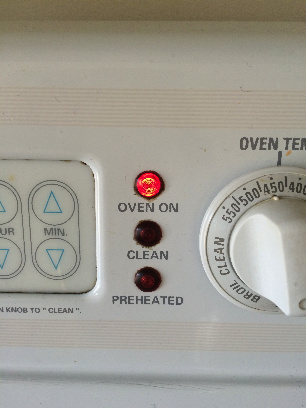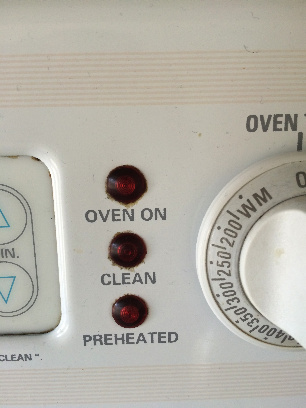1. OpenCV 3.0
2. Python 2.7
3. Numpy 1.9

## 步骤

### 加载需要的包

1. argparse —— 参数处理库。
2. numpy —— 高度优化的数值运算库。OpenCV 在数组结构中使用 numpy。
3. cv2 —— OpenCV 中图像处理库。
``````
import argparse
import numpy as np
import cv2``````

### 载入图片

``````

### 图片降噪

``````
blur_image = cv2.medianBlur(image, 3)``````

### 把图片颜色转为 HSV 格式

HSV —— 色度、饱和度和纯度（亮度）。HSV 可以让我们提取出一个彩色对象，因为它比 BGR 格式（译者注：与我们常说的RBG色彩模型类似，三个字母分别代表红蓝绿三色）更容易表征颜色。

``````
hsv_image = cv2.cvtColor(blur_image, cv2.COLOR_BGR2HSV)``````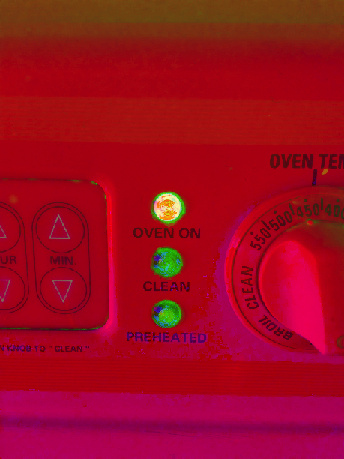### 检测图片中的颜色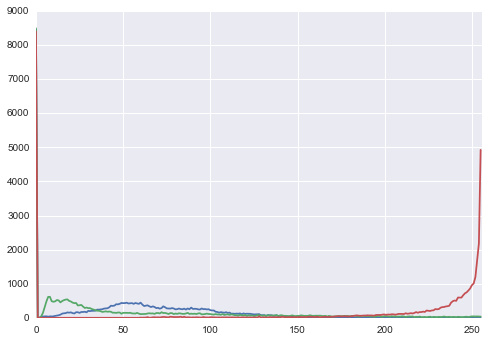``````
lower = np.array(lower_color, np.uint8)
upper = np.array(upper_color, np.uint8)

# Create a mask from the colors
return output_image

# Get lower red hue
lower_red_hue = create_hue_mask(hsv_image, [0, 100, 100], [10, 255, 255])

# Get higher red hue
higher_red_hue = create_hue_mask(hsv_image, [160, 100, 100], [179, 255, 255])``````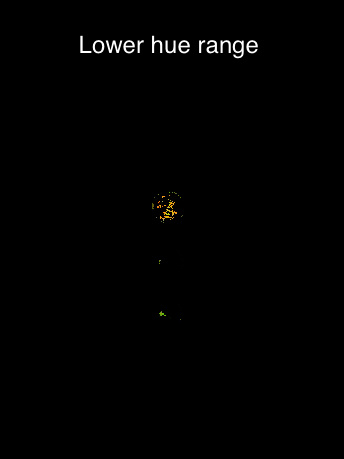``````
full_image = cv2.addWeighted(lower_red_hue, 1.0, higher_red_hue, 1.0, 0.0)``````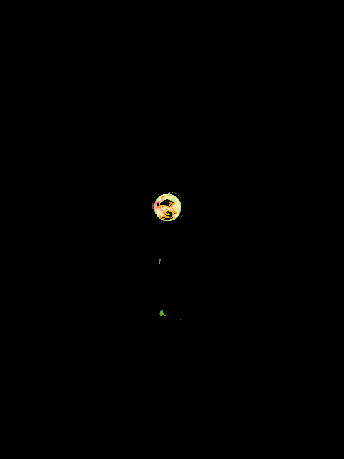### 发现图片中的圆圈

1. 灰度图输入。
3. 累加器和图片分辨率的反比。在本例中，为1.2。
4. 待检测圆圈圆心的最小距离，本例中为100。
``````
#Convert image to grayscale
image_gray = cv2.cvtColor(full_image, cv2.COLOR_BGR2GRAY)
#Find circles in the image

circles = cv2.HoughCircles(image_gray, cv2.HOUGH_GRADIENT, 1.2, 100)``````

## 结果

``````
# Draw the circles on the original image
circles = np.round(circles[0, :]).astype("int")
for (center_x, center_y, radius) in circles:
cv2.circle(image, (center_x, center_y), radius, (0, 255, 0), 4)``````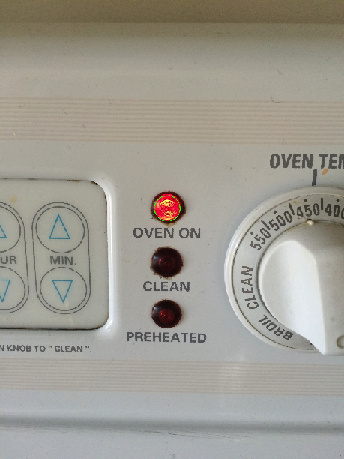## 下一步

1. 检测特定灯的开启，用以了解烤箱的真实状态。
2. 建立一个服务以便远程检查烤箱状态。
3. 把该功能加入树莓派（译者注：基于Linux的迷你开发板），我们就拥有可以警告烤箱关闭与否的小型设备。

Python-OpenCV 处理视频（一）： 输入输出
Python-OpenCV 处理视频（二）： 视频处理
Python-OpenCV 处理视频（三）： 标记运动轨迹
Python-OpenCV 处理视频（四）： 运动检测
Python-OpenCV 处理视频（五）：运动方向判断
Python-OpenCV 杂项（一）：图像绘制
Python-OpenCV 杂项（二）： 鼠标事件
Python-OpenCV 杂项（三）： 程序性能的检测和优化
Python-OpenCV 处理图像（六）：对象识别
Python-OpenCV 处理图像（七）：图像灰度化处理